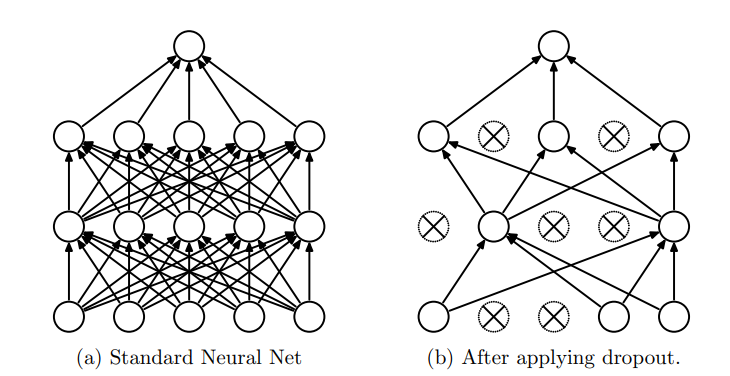# Drouout起源

• 捕捉高阶特征依赖，容易过拟合
• 训练费时费力

# 什么是Dropout

Dropout是一项技术可以解决上面提到的问题，它可以理解是一种正则化的技术，它的原理是在模型正向传播过程中，以一定的概率p使隐层中的神经元暂时性失活，注意只是暂时失活，并不是永久失活，每个mini-batch进行传播时，都会随机失活一定神经元，这样就会缓解过拟合的现象，原因是训练每个mini-batch时，并不是所有的神经元都会参与训练，说白了就是每个传播都是网络结构的部分神经元在发挥作用，这样与原网络就会少很多训练参数，因为失活一定神经元，会导致每个mini-batch的数据不会完全拟合，这就是Dropout的原理所在。• 训练： p x ∗ 1 + ( 1 − p ) ∗ 0 = p x px*1+(1-p)*0=px
• 推断： x x

• 方法一： x / = p x/=p
• 方法二： x ∗ = p x*=p

# 代码实现

import numpy as np

"""
"""
raise ValueError('Dropout prob must be in interval [0,1].')

seed = np.random.seed(seed)

random_tensor = np.random.binomial(n=1, p=keep_prob, size=x.shape)

x *= random_tensor

x /= keep_prob

return x

x = np.random.uniform(low=1, high=5, size=10)